# FTCE: Algebraic Thinking Chapter Exam

Exam Instructions:

Choose your answers to the questions and click 'Next' to see the next set of questions. You can skip questions if you would like and come back to them later with the yellow "Go To First Skipped Question" button. When you have completed the practice exam, a green submit button will appear. Click it to see your results. Good luck!

### Page 1

#### Question 4 4. The equation for the following graph could be _____.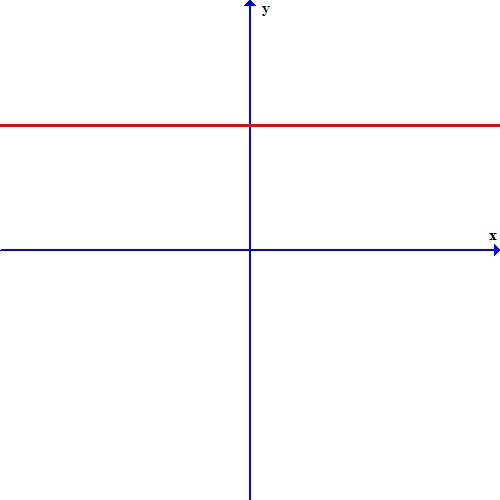### Page 2

#### Question 6 6. For these questions, I strongly recommend using either the CLEP calculator (found online) or one you use in class. Find x.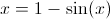#### Question 8 8. For these questions, I strongly recommend using either the CLEP calculator (found online) or one you use in class. Find x.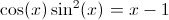#### Question 9 9. If the graph below has the equation Ax + By = -4, what must be true about A and B?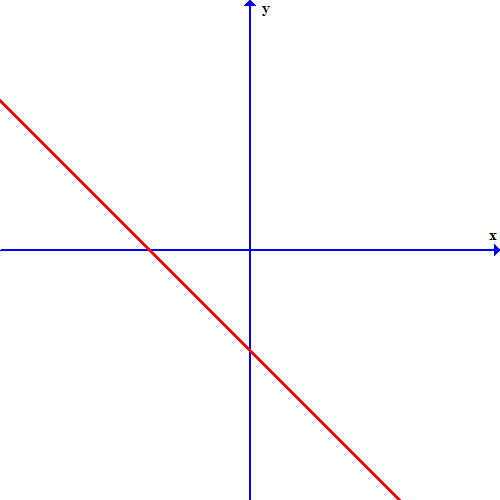### Page 3

#### Question 15 15. For these questions, I strongly recommend using either the CLEP calculator (found online) or one you use in class. Find x.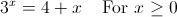### Page 4

#### Question 16 16. What part of this graph is the solution area?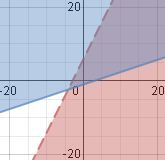#### Question 19 19. This graph corresponds to which inequality?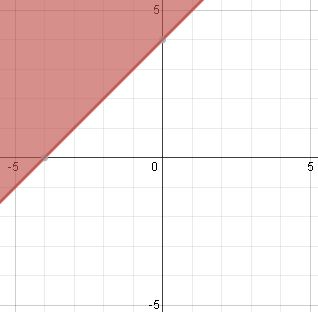### Page 5

#### Question 23 23. For these questions, I strongly recommend using either the CLEP calculator (found online) or one you use in class. Where is the maximum value of f(x) between 0 and pi?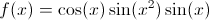### Page 6

#### Question 27 27. What is the slope-intercept form equation of the graph below?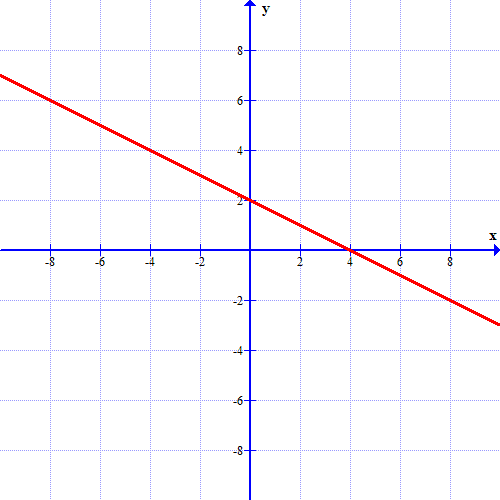#### Question 29 29. In the sequence 9, 14, 19, 24, 29,.... Say we're using a to describe the terms. What is the value of the term below?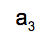#### Question 30 30. What is the equation of the graph below?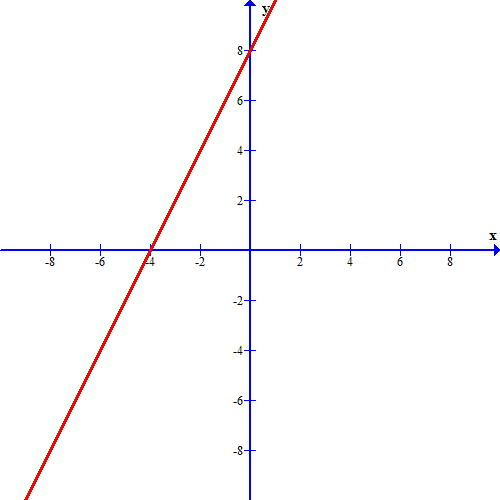#### FTCE: Algebraic Thinking Chapter Exam Instructions

Choose your answers to the questions and click 'Next' to see the next set of questions. You can skip questions if you would like and come back to them later with the yellow "Go To First Skipped Question" button. When you have completed the practice exam, a green submit button will appear. Click it to see your results. Good luck!

Support